# 1. - 18 points SerCP11 15..P.039. My Notes Ask Your Teache Refer to the figure below....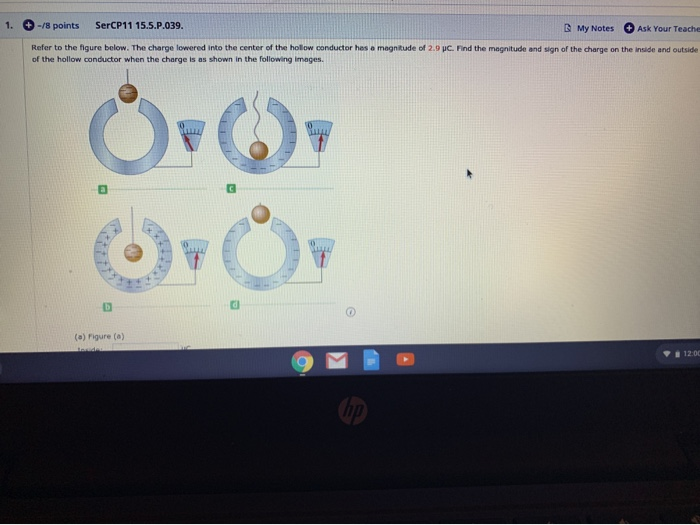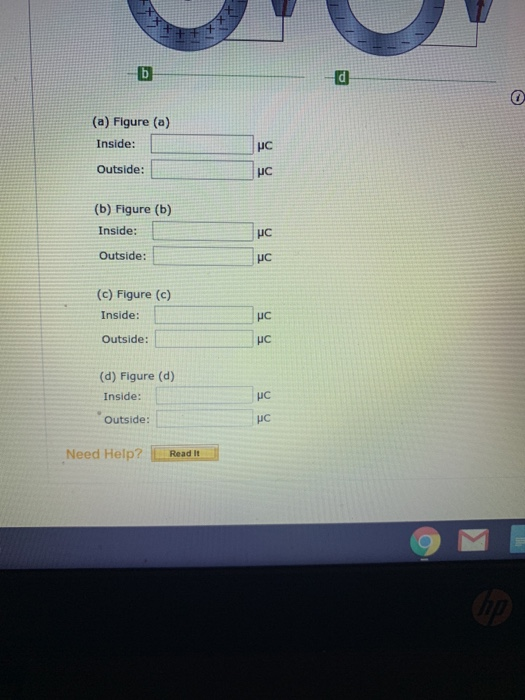1. - 18 points SerCP11 15..P.039. My Notes Ask Your Teache Refer to the figure below. The charge lowered into the center of the hollow conductor has a magnitude of 2.9 p. Find the magnitude and sign of the charge on the inside and outside of the hollow conductor when the charge is as shown in the following images. (a) Figure(a) 120
(a) Figure (a) Inside: Outside: (b) Figure (b) Inside: Outside: (c) Figure (c) Inside: Outside: (d) Figure (d) Inside: Outside: Need Help? Read It

(a)

The charge is not in contact with hollow conductor , hence

Qinside = 0

Qoutside = 0

b)

due to negative charge on the hanging charge, an equal amount of positive charge is induced on he inner surface of the conductor. So

Qinside = 2.9 C

and equal amount of negative charge goes away to the surface to keep the hollow conductor neutral.

Qoutside = - 2.9 C

c)

Due to transfer of charge, all the charge goes to the surface of hollow conductor, hence

Qinside = 0 C

Qoutside = - 2.9 C

d)

The charge remains undisturbed on the surface of conductor, hence

Qinside = 0 C

Qoutside = - 2.9 C

Similar Homework Help Questions
• ### Refer to the figure below. The charge lowered into the center ofthe hollow conductor has a magnitude of 5 µC. Find the m...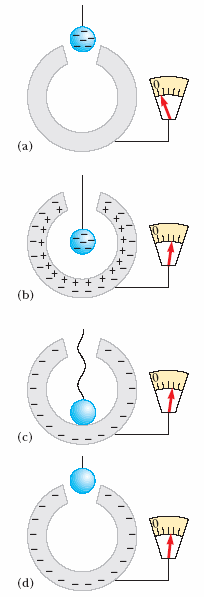Refer to the figure below. The charge lowered into the center ofthe hollow conductor has a magnitude of 5 µC. Find the magnitude and sign of thecharge on the inside and outside of the hollow conductor when thecharge is as shown in the following images: (a) Figure (a) Inside: 1 Your response differs from the correct answerby orders of magnitude. µC Outside: 2 Your response differs from the correct answerby orders of magnitude. µC (b) Figure (b) Inside: 3 µC...

• ### SerCP11 22.7.0P.039 Ask Your Teache 5. -/1 points My Notes A technician directs a ray of...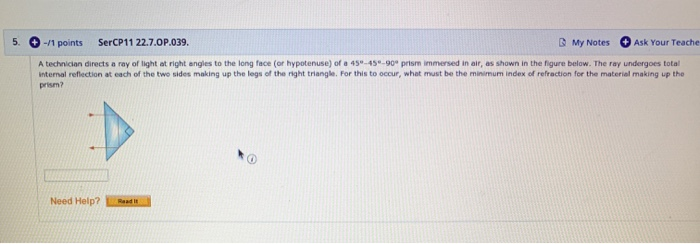SerCP11 22.7.0P.039 Ask Your Teache 5. -/1 points My Notes A technician directs a ray of light at right angles to the long face (or hypotenuse) of a 45-45-90 prism immersed in air, as shown in the figure below. The ray undergoes total internal reflection at each of the two sides making up the legs of the right triangle. For this to occur, what must be the minimum index of refraction for the material making up the prism? Need Help?...

• ### + -12 points SerCP11 15.3.P.020 My Notes Ask Your Teache An electron is accelerated by a...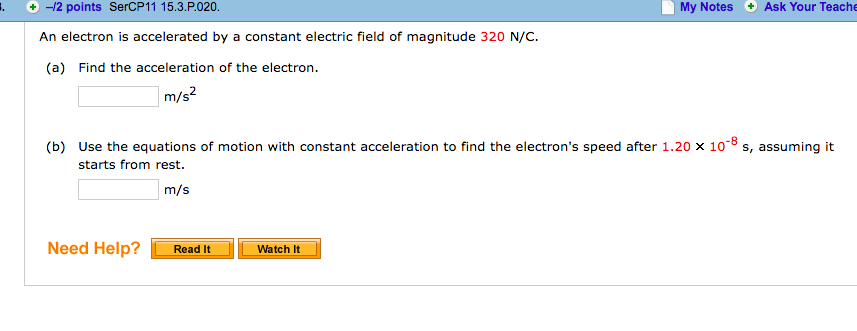+ -12 points SerCP11 15.3.P.020 My Notes Ask Your Teache An electron is accelerated by a constant electric field of magnitude 320 N/C. (a) Find the acceleration of the electron. m/s Use the equations of motion with constant acceleration to find the electron's speed after 1.20 x 10-8 s, assuming it starts from rest. (b) m/s Need Help?Read It

• ### 4. 0/6 points | Previous Answers SerCP11 15.2.P.010. My Notes Ask Your Teache Calculate the magnitude...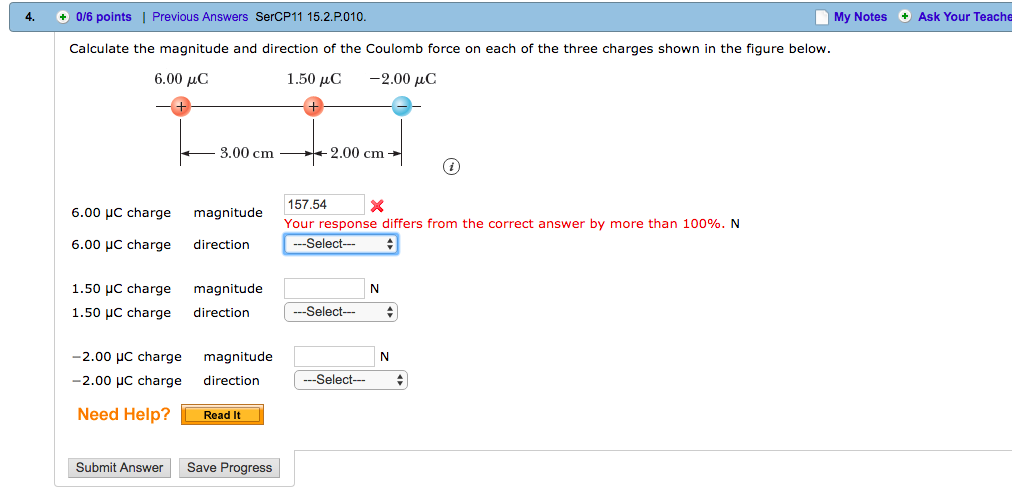4. 0/6 points | Previous Answers SerCP11 15.2.P.010. My Notes Ask Your Teache Calculate the magnitude and direction of the Coulomb force on each of the three charges shown in the figure below. 6.00 AC 1.50 μC -2.00 μC 3.00 cm2.00 cm 157.54X Your response differs from the correct answer by more than 100%. N 6.00 charge magnitude 6.00 HC charge direction 1.50 HC charge magnitude -Select 1.50 uC charge direction --Select- -2.00 μc charge magnitude IN -2.00 HC charge...

• ### Charge in conductor - help pleaassseeeRefer to Figure 15.20. The charge lowered into the center of thehollow conductor has a magnitude of 5µC. Find the magnitude and sign of the charge on the insideandoutside of the hollow conductor when the charge is as shown inthe following image.Figure 15.20(a) Figure 15.20aInside: µCOutside: µC(b) Figure 15.20bInside: µCOutside: µC(c) Figure 15.20cInside: µCOutside: µC(d) Figure 15.20dInside: µCOutside: µC

• ### -12 points SerCP11 20.5.P.038. My Notes Ask Your Teache A solenoid with 515 turns has a...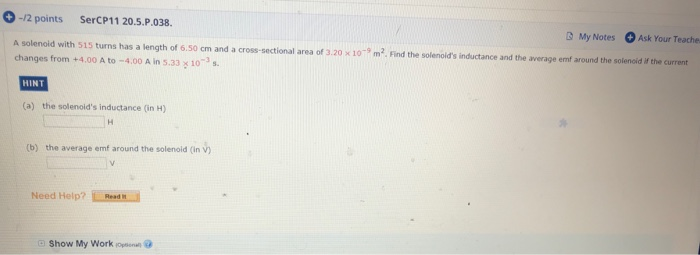-12 points SerCP11 20.5.P.038. My Notes Ask Your Teache A solenoid with 515 turns has a length of 6.50 cm and a cross-sectional area of 3.20 x10 m. Find the solenoid's inductance and the average emf around the solenoid if the current changes from+4.00 A to-4.00 A in 5.33 x 10 s HINT (a) the solenoid's inductance (in H) H (b) the average emf around the solenoid (in V) V Need Help? Read I Show My Work opena

• ### 9. О-t1 points SerCP10 15.PO37 My Notes Ask Your Teache If the electric field strength in...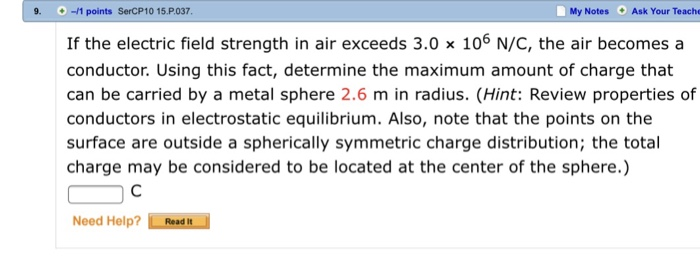9. О-t1 points SerCP10 15.PO37 My Notes Ask Your Teache If the electric field strength in air exceeds 3.0 x 106 N/C, the air becomes a conductor. Using this fact, determine the maximum amount of charge that can be carried by a metal sphere 2.6 m in radius. (Hint: Review properties of conductors in electrostatic equilibrium. Also, note that the points on the surface are outside a spherically symmetric charge distribution; the total charge may be considered to be located...

• ### 7. + -/1 points SerPSE 10 24.A.P.047. My Notes Ask Your Teach For the configuration shown...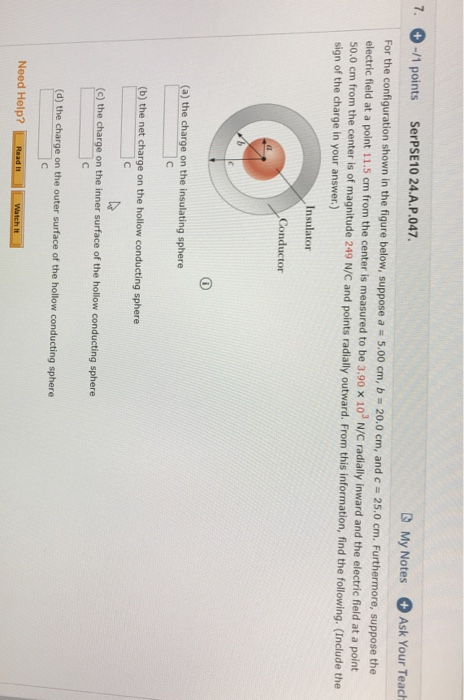7. + -/1 points SerPSE 10 24.A.P.047. My Notes Ask Your Teach For the configuration shown in the figure below, suppose a = 5.00 cm, b = 20.0 cm, and c = 25.0 cm. Furthermore, suppose the electric field at a point 11.5 cm from the center is measured to be 3.90 X 10' N/C radially inward and the electric field at a point 50.0 cm from the center is of magnitude 249 N/C and points radially outward. From this...

• ### 12. [-/2.5 Points] DETAILS SERCP11 1.7.P.039.MI. MY NOTES ASK YOUR TEACHER PRACTICE ANOTHER A point is...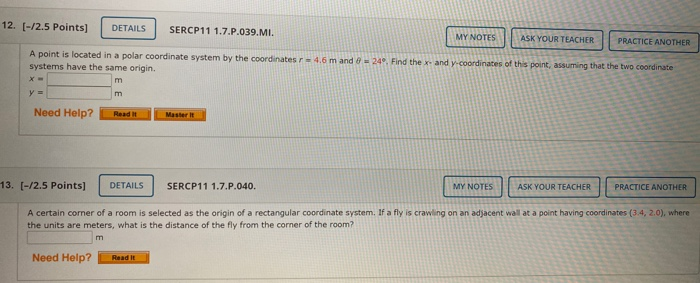12. [-/2.5 Points] DETAILS SERCP11 1.7.P.039.MI. MY NOTES ASK YOUR TEACHER PRACTICE ANOTHER A point is located in a polar coordinate system by the coordinates r - 4.6 m and 8 - 24. Find the x- and y-coordinates of this point, assuming that the two coordinate systems have the same origin. m y = וח Need Help? Master it 13. [-/2.5 Points] DETAILS SERCP11 1.7.P.040. MY NOTES ASK YOUR TEACHER PRACTICE ANOTHER A certain corner of a room is selected...

• ### 1. /1 points SerCP11 15 A P.066. My Notes Ask Your Teacher Two small beads having...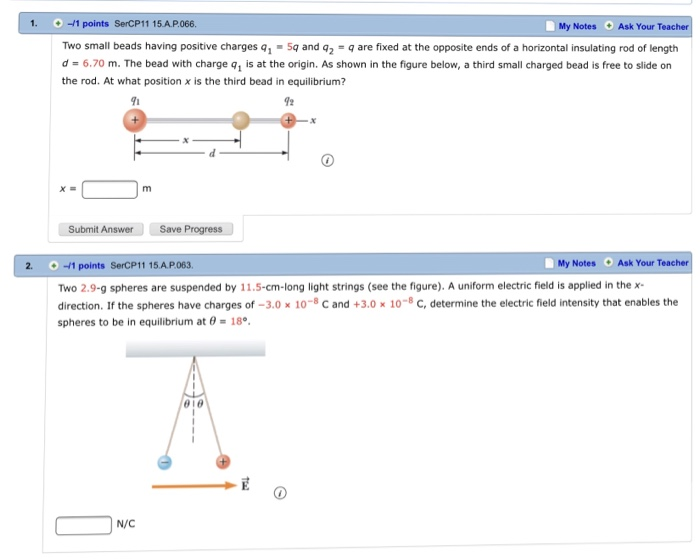1. /1 points SerCP11 15 A P.066. My Notes Ask Your Teacher Two small beads having positive charges qi·Sq and q2 = q are fixed at the opposite ends of a horizontal insulating rod of length d = 6.70 m. The bead with charge q1 is at the origin. As shown in the figure below, a third small charged bead is free to slide on the rod. At what position x is the third bead in equilibrium? 91 92 x=...

Free Homework App